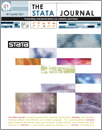Home  >>  Archives  >>  Volume 5 Number 2  >>  gr0012

#### The Stata JournalVolume 5 Number 2: pp. 259-273

Subscribe to the Stata Journal## Speaking Stata: Density probability plots

 Nicholas J. Cox Durham University, UK n.j.cox@durham.ac.uk
Abstract.   Density probability plots show two guesses at the density function of a continuous variable, given a data sample. The first guess is the density function of a specified distribution (e.g., normal, exponential, gamma, etc.) with appropriate parameter values plugged in. The second guess is the same density function evaluated at quantiles corresponding to plotting positions associated with the sample’s order statistics. If the specified distribution fits well, the two guesses will be close. Such plots, suggested by Jones and Daly in 1995, are explained and discussed with examples from simulated and real data. Comparisons are made with histograms, kernel density estimation, and quantile–quantile plots.

View all articles by this author: Nicholas J. Cox

View all articles with these keywords: density probability plots, distributions, histograms, kernel density estimation, quantile–quantile plots, statistical graphics